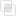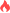python绘制散点图的两种方法

python散点图2021-09-24 09:45:341916浏览 · 0收藏 · 0评论1、调用scatter()函数，调用scatter()从给出的一堆随机点(包括x,y坐标)中绘制散点图。它可以单独控制每个散点与数据的匹配，使每个散点具有不同的属性。

2、另一种是调用plot()函数。

# -*- coding: utf-8 -*-
#By:Eastmount CSDN
import numpy as np
import matplotlib.pyplot as plt

#构造数据
x = np.random.randn(200)
y = np.random.randn(200)
print(x[:10])
print(y[:10])

#绘制散点图
plt.scatter(x, y)
plt.show()
numpy中有一些用来产生随机数的常用函数，randn()和rand()就属于其中。

numpy.random.randn(d0, d1, …, dn)：从标准正态分布中返回一个或多个样本值。
numpy.random.rand(d0, d1, …, dn)：产生随机样本，并且数字位于[0, 1]中。python for语句的应用场景python如何模拟用户自动打卡python逻辑取反的实现python Pytest有什么特点python如何打印矩阵Python Modules是什么意思python三种导入模块的方式python查找计算函数的整理python有哪些转换字符串的函数python填充压缩的函数总结python分割拼接函数的介绍python判断字符串函数的归纳python如何实现图像等比缩放python setup和teardown的使用js事件委托如何理解8228

js中hasOwnProperty的属性用法7373